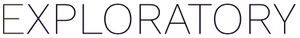# Using ifelse with a parameter

#1

The goal here is to double the flow_mc column if the “M” (municipal) permit type is selected:

When I use this statement:

it sort of works, but it only uses the value for the first row! How can I get the output to vary by row?

Thanks in advance``````24. Create Calculation (Mutate)' step has an error. Error : Problem with `mutate()` input `calculation_1`. x `true` must be length 1 (length of `condition`), not 1040. i Input `calculation_1` is `if_else(exploratory_env\$Permit_Type == "M", flow_mc * 2, flow_mc).
``````

FYI I tried two different work arounds:

1. creating a new column for the calculation(same result) and
2. using “if_else” instead, which returned the above error.

And I can confirm that when I leave out the conditional statement, it works as expected:

Update: well maybe parameters don’t work this way? I was able to come up with a work-around that used a join and the “Replace by Setting Conditions” tool and it worked great!

#2

This problem seems to be caused by the following two issues.

• Exploratory parameters are treated as scalars(Single value).
• The `ifelse(test, yes, no)` function returns the result of the decision by the number of the vector size passed as the test argument.

As a result of all this, the `ifelse` function in the `mutate` function is supposed to return an error.

If you expand the Exploratory parameter by the number of records in the data frame as shown below, it will work fine.

``````# make 2 cols in mutate function

# to expand from scalar parameter to vector
permit_type = @{permit_type}

## if you override col, set same col name
value_times10 = ifelse(permit_type == 'M', value*10, value)
``````

The above tasks can be summarized and described as follows

``````ifelse(rep(@{permit_type},n()) == 'M', value*10, value)
``````

If permit_type is not “M”, the value will not be multiplied.

If permit_type is “M”, value will be multiplied.

I apologize if my English is not good enough to properly understand the intent of your question.

Is this likely to solve your problem?

2 Likes
How to impute <NA> values in different categories?
#3

@sugiaki This is fantastic. Thank you so much for the thorough explanation and the excellent solution!

1 Like